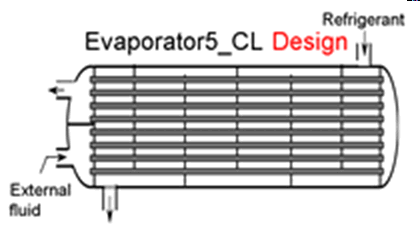Contents - index

Evaporator5_CLEvaporator5_CL determines the required heat transfer surface area to evaporate a two-phase refrigerant to a specified degree of superheat by heat exchanger with an external fluid.  Thermal energy is transferred to an external fluid, which may be a liquid such as water or a brine.  It is necessary to provide the heat transfer coefficients on the external fluid side of the heat exchanger and for the saturated and superheated sections on the refrigerant side.  These heat transfer coefficients can be determined using the functions in the Heat Transfer Library, as illustrated in the example below.

This library file can be used in the design stage of the evaporator by inputing the design inlet conditions to determine the required evaporator size.  Use Evaporator6_CL to determine the refrigerant and external fluid outlet states for given inlet conditions and evaporator area.

Inputs:

R\$:   name of the refrigerant

m_dot_R:  refrigerant flow rate (kg/s, lbm/hr)

h_R_in:  specific enthalpy of entering refrigerant (J/kg, kJ/kg, Btu/lbm)

DELTAT_sh:  number of degrees of superheating (C, K, F, R)

P_R:  refrigerant pressure (Pa, kPa, bar, MPa, psia, atm)

Config\$: a string constant or string variable that must be either 'counterflow' or 'parallelflow'

m_dot_fluid:  mass flow rate of the external fluid  (kg/s, lbm/hr)

T_fluid_in:  external fluid inlet temperature  (C, K, F, R)

cp_fluid:  specific heat of external fluid (J/kg-K, kJ/kg-K, Btu/lbm-R)

h_fluid:  effective outside (external fluid) heat transfer coefficient per unit refrigerant tube area (W/m^2-K, Btu/hr-ft^2-R)

A_fluid\A_R:  ratio of effective heat transfer area on the external fluid side to the heat transfer area for the refrigerant*

h_R_sat:  average heat transfer coefficient for refrigerant in the saturated regime  (W/m^2-K, Btu/hr-ft^2-R)

h_R_sh:  average heat transfer coefficient for refrigerant in the superheated regime  (W/m^2-K, Btu/hr-ft^2-R)

Outputs:

A_R:  required heat transfer area for the refrigerant (m^2, ft^2)

UA:  overall heat transfer coefficient (W/K ,Btu/hr-R)

Q_dot:  overall heat transfer rate to evaporator (W, kW, Btu/hr)

h_R_out refrigerant outlet specific enthalpy (J/kg, kJ/kg, Btu/lbm)

T_fluid_out:  outlet temperature of the external fluid  (C, K, F, R)

f_sh:  fraction of the evaporator area that is used for desuperheating

*A_fluid\A_R is the ratio of the product of the overall fin efficiency and total (finned and unfinned) area on the external fluid side to the total surface area inside the refrigerant flow passages.  For unfinned surfaces, A_fluid\A_R is the ratio of the outer surface to inner surface areas.

Notes:

In actual operation, the heat exchanger type may shell-in-tube, plate or other type.  However, Config\$ may only be set to 'Parallelflow' or 'Counterflow'.  These two options provide upper and lower bounds on the required heat transer area.

Example:

\$UnitSystem SI C kPa kJ mass

R\$='R134a' "refrigerant"

m_dot_R=0.08 [kg/s] "refrigerant flow rate"

T_cond_out=38 [C]  "exit temperature of refrigerant from condenser"

P_cond=1 [MPa]*convert(MPa,kPa)  "condenser pressure"

h_cond_out=enthalpy(R\$,T=T_cond_out,P=P_cond)  "specific enthalpy of refrigerant exiting condenser"

P_R=200 [kPa]  "evaporator pressure"

T_R_sat=temperature(R\$,P=P_R,x=0)  "evaporator saturation temperature"

h_R_in=h_cond_out  "specific enthalpy of refrigerant entering evaporator"

x_R_in=quality(R\$,h=h_R_in,P=P_R)  "inlet quality"

Fluid\$='EG' "ethylene glycol-water is the external fluid"

Conc=30 [%] "concentration of the ethylene glycol - water solution"

T_fluid_in=5 [C]  "inlet temperature of external fluid"

Config\$='counterflow'  {parallelflow} "heat exchanger configuration;  counterflow and parallelflow bound the problem"

m_dot_fluid=0.5 [kg/s] "mass flow rate of external fluid"

cp_fluid=cp(EG,T=T_fluid_in,C=Conc) "specific heat of external fluid"

DELTAT_sh=5 [DELTAK]  "degrees of superheat"

h_R_sh=205 [W/m^2-K]  "convection coefficient for superheated R134a."

h_R_sat=540 [W/m^2-K]  "convection coefficient for saturated R134a - see calculation of h_cond below"

h_fluid=475 [W/m^2-K]  "convection coefficient for external fluid  "

A_fluid\A_R=1.186 "ratio of area on the external fluid side to that on the refrigerant side"

Call evaporator5_cl(R\$, m_dot_R, h_R_in, P_R, DELTAT_sh, Config\$, m_dot_fluid, T_fluid_in, cp_fluid, h_fluid, A_fluid\A_R,  h_R_sat, h_R_sh :  A_R, UA, Q_dot, h_R_out, T_fluid_out, f_sh)

{!__________________________________________________________________________}

"!Calculation of the heat transfer coefficients using the Heat Transfer Library functions"

N_circuits=12  "number of parallel circuits"

D_shell=0.175 [m]  "diameter of shell"

W=0.75 [m]   "length of tubes"

N_tubes=round(A_R/(pi*W*D_in))  "required number of tubes"

D_o=1.15 [cm]*convert(cm,m)  "outside tube diameter"

th = 0.9 [mm]*convert(mm,m)   "tube wall thickness"

D_in=D_o-2*th   "inner tube diameter"

A_fluid\A_R1=D_o/D_in  "ratio of heat transfer area on external fluid side to that on refrigerant side"

"Determine heat transfer coefficients for the external fluid on the outside and refrigerant on the inside of the tubes"

Area_shell=pi*D_shell^2/4  "cross-sectional area of the heat exchanger shell"

Area_tubes=N_tubes*pi*D_o^2/4  "cross-sectional area of the refrigerant tubes"

Area_fluid=Area_shell-Area_tubes  "free flow area for external fluid"

vel=m_dot_fluid/density(Fluid\$,T=T_fluid_in,C=Conc)/Area_fluid  "velocity of external fluid"

"calculate heat transfer coefficient for the external fluid in a tube bank"

Pitch=D_o*1.2

G\$='SQUARE'

D_hp=4*(Area_shell-Area_tubes)/(pi*N_tubes*D_o+pi*D_shell)

Call tube_bundle(Fluid\$, T_fluid_in, Conc, G\$, Vel, D_o, Pitch, T_R_sat, W, D_hp:DELTAP_fluid, h_fluid1, Re, ,  )

"calculate the heat transfer coefficients for the refrigerant in the saturated and superheat regimes"

L_sh=N_tubes/N_circuits*f_sh*W

Call pipeflow(R\$,T_R_sat+DELTAT_sh, P_R, m_dot_R/N_circuits, D_in, W, 0: h_T_sh, , DELTAP_sh,  ,  , ) "superheat section"

G=m_dot_R/(pi*D_in^2/4)/N_circuits "mass flux"

q``=Q_dot/A_r*convert(kW,W) "heat flux"

h_evap=flow_boiling_avg(R\$, T_R_sat, G, D_o, x_R_in,1, q``, 'Horizontal') "saturated section"

{!___________________________________________________________________________}

{Solution

Area_fluid=0.007642 [m^2]

Area_shell=0.02405 [m^2]

Area_tubes=0.01641 [m^2]

A_fluid\A_R=1.186 [-]

A_fluid\A_R1=1.186

A_R=3.605 [m^2]

Conc=30 [%]

Config\$='counterflow'

cp_fluid=3.673 [kJ/kg-K]

DELTAP_fluid=0.1438 [kPa]

DELTAP_sh=0.6333 [kPa]

DELTAT_sh=5 [DELTAK]

D_hp=0.004884 [m]

D_in=0.0097 [m]

D_o=0.0115 [m]

D_shell=0.175 [m]

Fluid\$='EG'

f_sh=0.05096

G=90.21 [kg/m^2-s]

G\$='SQUARE'

h_cond_out=105.3 [kJ/kg]

h_evap=541.8 [W/m^2-K]

h_fluid=475 [W/m^2-K]

h_fluid1=472 [W/m^2-K]

h_R_in=105.3 [kJ/kg]

h_R_out=248.7 [kJ/kg]

h_R_sat=540 [W/m^2-K]

h_R_sh=205 [W/m^2-K]

h_T_sh=204.8 [W/m^2-K]

L_sh=0.5032 [m]

m_dot_fluid=0.5 [kg/s]

m_dot_R=0.08 [kg/s]

N_circuits=12

N_tubes=158

Pitch=0.0138 [m]

P_cond=1000 [kPa]

P_R=200 [kPa]

Q_dot=11.47 [kW]

q``=3183 [W/m^2]

R\$='R134A'

Re=176.2

th=0.0009 [m]

T_cond_out=38 [C]

T_fluid_in=5 [C]

T_fluid_out=-1.248 [C]

T_R_sat=-10.09 [C]

UA=970.8 [W/K]

vel=0.06271 [m/s]

W=0.75 [m]

x_R_in=0.3245

}